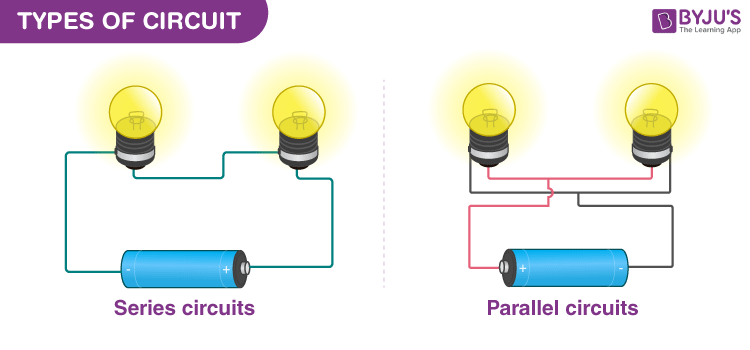# Types of Circuits

An electrical circuit consists of an organization of elements for the storage, transmission and conversion of energy. Energy enters a circuit through one or more sources and leaves through one or more sinks. In the sources energy is transformed from the thermal, chemical, electromagnetic or mechanical form into electrical form; in the sinks a reverse procedure takes place. In an electrical circuit, energy is transported through the agency of electrical charge and through the medium of magnetic and electric fields. There are different types of circuits, parallel and series circuits.## Series Circuits

A series circuit is one in which numerous resistances are linked one after the other. Such connection is also termed as an end to end connection or a cascade connection. There is a single path for the flow of current.

### Properties of Series circuit:

• The same current travels through every resistance.
• The supply voltage V is the total of the individual voltage dips across the resistances.

V = V+ V+ V+ …..+ Vn

• The equivalent electrical resistance is equivalent to the total of the individual resistances.
• The equivalent resistance is the biggest of all the individual resistances.

R > R1, R > R2,….., R > Rn

### Parallel Circuits

The parallel circuit is one in which numerous resistances are linked across one another in such a manner that one terminal of every resistance is connected to form a junction point while the remaining end is also linked to form another point.

### Properties of Parallel Circuits:

• A similar potential difference gets across all the resistances in parallel.
• The total current gets distributed into the number of paths equivalent to the number of resistances in parallel. The aggregate current is always the summation of all the individual currents.

I = I+ I+ I+ ……+ In

• The reciprocal of the equivalent resistance of a parallel circuit is equivalent to the sum of the reciprocal of the individual resistances.
• The equivalent resistance is the minutest of all the resistances.

R < R1, R < R2, ….., R < Rn

• The equivalent conductance is the mathematical addition of the single conductances.

The equivalent resistance is lesser than the smallest of all the resistances linked in parallel.

### Dissimilarities

The variances between series and parallel circuits are demonstrated in the table underneath.

#### Circuit In Parallel

There is a single current pathway There are multiple current pathways
All components have similar current running through them All components have similar potential difference across them
The sum of the potential dips across each component is equivalent to the emf of the source. The sum of the currents flowing into any point in the circuit is equivalent to the sum of the currents flowing out of that point.

## Watch the video and solve important questions in the chapter Electricity Class 10Stay tuned to BYJU’S and Fall in Love with Learning!

Test your Knowledge on Types Of Circuits